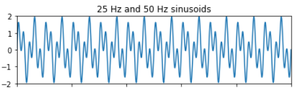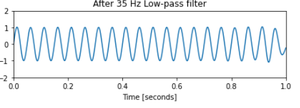Noise Removal using Lowpass Digital Butterworth Filter in Scipy – Python

• Last Updated : 13 Jan, 2021

In this article, the task is to write a Python program for Noise Removal using Lowpass Digital Butterworth Filter.

What is the noise?

Noise is basically the unwanted part of an electronic signal. It is often generated due to fault in design, loose connections, fault in switches etc.

What to do if we have noise in our signal?

To remove unwanted signals/noise we use filters of different types and specifications. Generally in the industry we need to choose the best fit by testing it with the signal to pinpoint the best filter to be used for removing the noise in a given use case.

What are we going to do now?

We are going to implement a Lowpass Digital Butterworth Filter now to remove the unwanted signal/noise of a combination of sinusoidal waves.

Filter Specifications:

• Signal made up of 25 Hz and 50 Hz
• Sampling frequency 1kHz.
• Order N=10 at 35Hz to remove 50Hz tone.

Step by Approach:

Step 1:Importing the libraries

Python3

 # import required libraryimport numpy as npimport scipy.signal as signalimport matplotlib.pyplot as plt

Step 2:Defining the specifications

Python3

 # Specifications of the filterf1 = 25  # Frequency of 1st signalf2 = 50  # Frequency of 2nd signalN = 10  # Order of the filter    # Generate the time vector of 1 sec durationt = np.linspace(0, 1, 1000)  # Generate 1000 samples in 1 sec  # Generate the signal containing f1 and f2sig = np.sin(2*np.pi*f1*t) + np.sin(2*np.pi*f2*t)

Step 3:Plot the original signal with noise

Python3

 # Display the signalfig, (ax1, ax2) = plt.subplots(2, 1, sharex=True)ax1.plot(t, sig)ax1.set_title('25 Hz and 50 Hz sinusoids')ax1.axis([0, 1, -2, 2])  # Design the Butterworth filter using # signal.butter and output='sos'sos = signal.butter(50, 35, 'lp', fs=1000, output='sos')

Output:Step 4:Plot of the signal after removing noise

Python3

 # Filter the signal by the filter using signal.sosfilt# Use signal.sosfiltfilt to get output inphase with inputfiltered = signal.sosfiltfilt(sos, sig)    # Display the output signalax2.plot(t, filtered)ax2.set_title('After 35 Hz Low-pass filter')ax2.axis([0, 1, -2, 2])ax2.set_xlabel('Time [seconds]')plt.tight_layout()plt.show()

Output:Step 5: Implementation

Python3

 # import required libraryimport numpy as npimport scipy.signal as signalimport matplotlib.pyplot as plt  # Givenf1 = 25  # Frequency of 1st signalf2 = 50  # Frequency of 2nd signalN = 10  # Order of the filter  # Generate the time vector of 1 sec duration# START CODE HERE ### (≈ 1 line of code)# Generate 1000 samples in 1 sect = np.linspace(0, 1, 1000)  # Generate the signal containing f1 and f2# START CODE HERE ### (≈ 1 line of code)sig = np.sin(2*np.pi*f1*t) + np.sin(2*np.pi*f2*t)  # Display the signalfig, (ax1, ax2) = plt.subplots(2, 1, sharex=True)ax1.plot(t, sig)ax1.set_title('25 Hz and 50 Hz sinusoids')ax1.axis([0, 1, -2, 2])  # Design the Butterworth filter using signal.butter and output='sos'# START CODE HERE ### (≈ 1 line of code)sos = signal.butter(50, 35, 'lp', fs=1000, output='sos')  # Filter the signal by the filter using signal.sosfilt# START CODE HERE ### (≈ 1 line of code)# Use signal.sosfiltfilt to get output inphase with inputfiltered = signal.sosfiltfilt(sos, sig)    # Display the output signalax2.plot(t, filtered)ax2.set_title('After 35 Hz Low-pass filter')ax2.axis([0, 1, -2, 2])ax2.set_xlabel('Time [seconds]')plt.tight_layout()plt.show()

Output:Attention geek! Strengthen your foundations with the Python Programming Foundation Course and learn the basics.

To begin with, your interview preparations Enhance your Data Structures concepts with the Python DS Course. And to begin with your Machine Learning Journey, join the Machine Learning - Basic Level Course

My Personal Notes arrow_drop_up